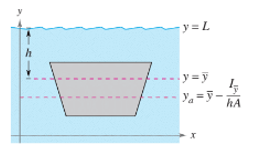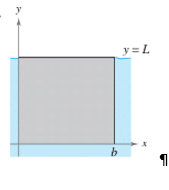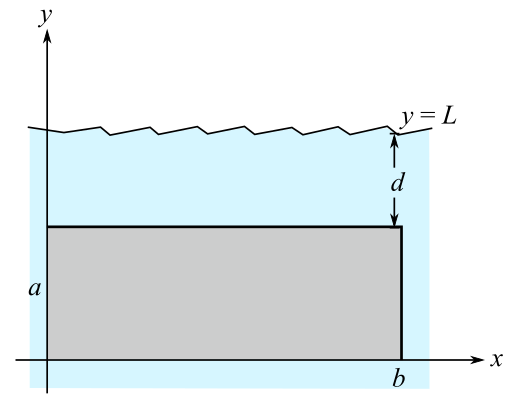Chapter 14.4, Problem 44E

Chapter
Section
Textbook Problem

Hydraulics In Exercises 43-46. determine the location of the horizontal axis y a at which a vertical gate in a dam is to be hinged so that there is no moment causing rotation under the indicated loading (see figure). The model for y a is y a = y ¯ − I y ¯ h A where y ¯ is the y-coordinate of the centroid of the gale, I y is the moment of inertia of the gate about the line y = y ¯ , h , h is the depth of the centroid below the surface, and A is the area of the gate.To determine

To calculate: The location on the horizontal axis, that is ya, at which the vertical gate in the dam is to be hinged to make sure there is no moment that leads to rotation under the loading indicated to in the given figure,Explanation

Given:

According to the given model, ya=y¯Iy¯hA, where y¯ represents the y-coordinate of the centroid of the gate, Iy¯ represents the moment of the inertia of the gate about the line that is written as y=y¯, h is used to denote the depth of the centroid below the surface, and A denotes the area of the gate.

Formula Used:

The moment of inertia is written as Iy=x1x2y1y2x2ρ(x,y)dydx, where x2 is used to denote the square of the distance from y-axis.

Calculation:

Have a look at the given figure,

The following can be interpreted from the given figure,

y¯=a2 when the length is represented by a and the centroid is at the center of the rectangle, as shown in the figure given below.

Have a look at another figure,

Still sussing out bartleby?

Check out a sample textbook solution.

See a sample solution

The Solution to Your Study Problems

Bartleby provides explanations to thousands of textbook problems written by our experts, many with advanced degrees!

Get Started

Other Equations Find all real solutions of the equation. 108. x3x44=0

Precalculus: Mathematics for Calculus (Standalone Book)

In Exercises 75-98, perform the indicated operations and/or simplify each expression. 81. (131+e)(131+e1)

Applied Calculus for the Managerial, Life, and Social Sciences: A Brief Approach

In Problems 19-44, factor completely. 38.

Mathematical Applications for the Management, Life, and Social Sciences

Are ABC and DEF congruent? Exercises 7, 8

Elementary Geometry For College Students, 7e